Next: 6.3 Formulation of the Up: 6.2 Lateral Discretization of Previous: 6.2.5 Truncation of the

## 6.2.6 Vector-Matrix Notation

Any further considerations are much simplified by switching over to a vector-matrix notation. For that we introduce the following four NODE/4-dimensional vectors comprising the (2Nx + 1) x (2Ny + 1) Fourier coefficients of the four relevant field components: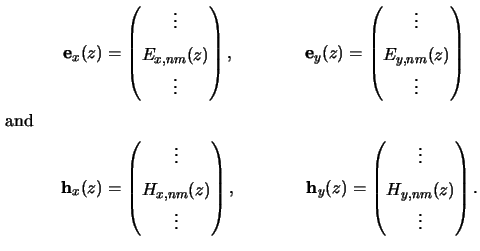Within each of these vectors the coefficients are arranged accordingly to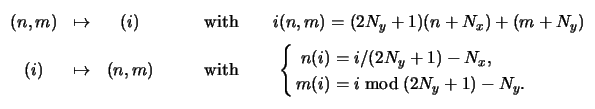(6.22)

The mapping is unique and invertible, i.e., any index pair (n, m) corresponds to one unequivocal vector-entry i, and vice versa.

The same methodology can be applied to the coefficients of the ODE system (6.22). Each of the eight sets rnm, pq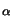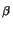(z) and gnm, pq(z) with (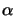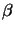)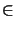{(xx),(xy),(yx),(yy)} is arranged in one NODE/4 x NODE/4-matrix. The ODE system can thus be written in the compact form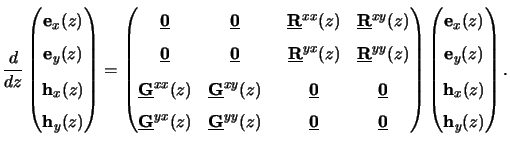(6.23)

The overall shape of the system matrix reflects the fundamental structure of the Maxwell equations since the derivative of the electric field depends only on the derivative of the magnetic field and vice versa.

Comprising the four field coefficient vectors of (6.30) to one NODE-dimensional vector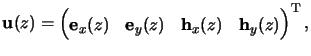(6.24)

we obtain the most compact and convenient formc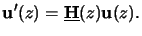(6.25)

Here the NODE x NODE-dimensional system matrix(z) follows from (6.34). Its entries hij(z) are determined by the Fourier coefficients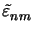(z) and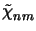(z) of the permittivity and its reciprocal, respectively. With the mapping (6.31) we obtain, e.g,(6.26)

whereby 1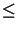i, j < NODE/4. The given example takes the explicit form (c.f. (6.22))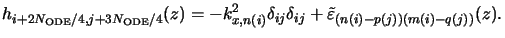(6.27)

#### Footnotes

... formc
A prime as superscript denotes derivation with respect to the independent variable that is the vertical coordinate z in our case.Next: 6.3 Formulation of the Up: 6.2 Lateral Discretization of Previous: 6.2.5 Truncation of the
Heinrich Kirchauer, Institute for Microelectronics, TU Vienna
1998-04-17